# Lions Weekly: March 30, 2022# A Note From Mr. Sandefur

Lion Family,

Springtime is a time when we see new birth all around us. From the budding flowers to the small animals that can now be found on our campus. This spring we are elated to share that we are also seeing new believers on our campus as several students have surrendered their lives to Christ recently in our chapel and small group settings. As the Apostle Paul writes in 2 Corinthians 5:17, these young men and women are new creations in Christ. While we celebrate all of the academic, athletic, and artistic achievements of our students, nothing brings greater joy than seeing students giving their life to Christ. Please join me in praying for these young men and women as they begin their faith journey.

Also, I want to encourage you to join me this weekend (Friday and Saturday) in support of our Fine Arts Department, as our students present The Sound of Music in Woody Auditorium. Our students and faculty have worked countless hours in preparation and would greatly appreciate your support at any of the three public shows. I look forward to seeing you as we support our student actors!

In Christ Alone,

Jeremy Sandefur
President

 table div table+table+table+table div table{width:100%;padding:0}table div table+table+table+table div table img{width:96.23%;padding:0;float:none}table div table+table+table+table div table td{width:100%;padding:0 1.88% 18px}/* styles */# Class of 2022

The King's Academy is pleased to announce the following students of the Class of 2022 who have been named this year’s Co-Valedictorians (statistical tie) and Salutatorian. These students have devoted countless hours of hard work and dedication to their studies to receive this time-honored recognition.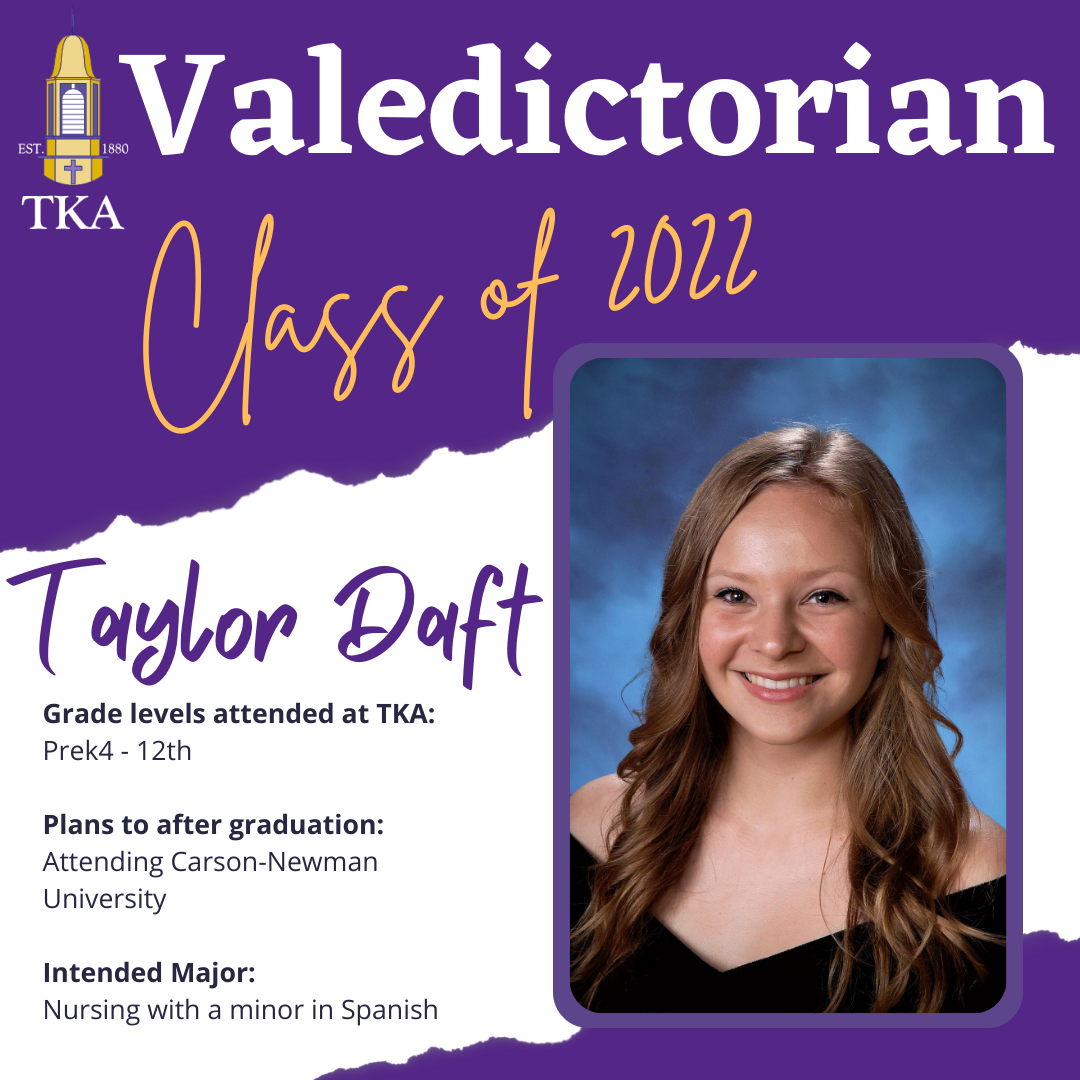table div table+table+table+table+table+table+table+table+table div table{width:100%;padding:0}table div table+table+table+table+table+table+table+table+table div table img{width:96.23%;padding:0;float:none}table div table+table+table+table+table+table+table+table+table div table td{width:100%;padding:0 1.88% 18px}/* styles */# This Weekend

TKA's Drama and Music Departments will be presenting The Sound of Music!

Tickets will be available at the door for \$10.00 per adult and \$5.00 per student.

- Friday, April 1, at 7:00 p.m.
- Saturday, April 2, at 1:00 p.m.
- Saturday, April 2, at 7:00 p.m.

 table div table+table+table+table+table+table+table+table+table+table+table div table{width:100%;padding:0}table div table+table+table+table+table+table+table+table+table+table+table div table img{width:96.23%;padding:0;float:none}table div table+table+table+table+table+table+table+table+table+table+table div table td{width:100%;padding:0 1.88% 18px}/* styles */# Monday, April 4

Jr. Beta is sponsoring a Crazy Hair Day to benefit the Isaiah 117 House. Bring your \$1.00 donation and help us Love Where We Live by helping the Isaiah 117 House.

 table div table+table+table+table+table+table+table+table+table+table+table+table+table div table{width:100%;padding:0}table div table+table+table+table+table+table+table+table+table+table+table+table+table div table img{width:96.23%;padding:0;float:none}table div table+table+table+table+table+table+table+table+table+table+table+table+table div table td{width:100%;padding:0 1.88% 18px}/* styles */Calling all 5th, 6th, and 7th grade boys! If you are interested in playing basketball this summer for team camp, tryouts are on Wednesday, April 6, in Tibbetts from 3:30 p.m. to 5:00 p.m.

 table div table+table+table+table+table+table+table+table+table+table+table+table+table+table+table div table{width:100%;padding:0}table div table+table+table+table+table+table+table+table+table+table+table+table+table+table+table div table img{width:96.23%;padding:0;float:none}table div table+table+table+table+table+table+table+table+table+table+table+table+table+table+table div table td{width:100%;padding:0 1.88% 18px}/* styles */# Prom Information

DATE: April 9, 2022
PLACE: Capitol Theatre, Maryville, TN
TIME: 6:30 pm - 10:30 pm

TICKETS: \$55 per person

Purchase tickets here

Desserts/Light Refreshments will be served.

ALL outside guests must be approved via the attached form.

DRESS CODE REQUIREMENTS:
Girls - Prom and homecoming dresses must be viewed on the individual in advance for suitable fitting - no exceptions. Skirts of the dresses may not be sheer (see through) material. “Dollar Bill” test will be used for the length. Length should be no shorter than width of dollar bill above knee (approximately two inches). Neckline and backline must be modest (backline must be above the natural waistline). Spaghetti straps and strapless styles are acceptable, if modest. The midriff should always remain covered. No open sides or sheer panels. No immodest tight dresses are allowed. Viewing dates will be provided for both homecoming and prom.

Boys - Suit & Tie or Tuxedo, Dress Shoes

Prom Dress Checks will be:
March 10 3:15-4:00
March 24 3:15-4:00
Or via email to lwallace@thekingsacademy.net and kpolk@thekingsacademy.net - send pics of front and back full length of dress in good lighting.

Rechecks April 1 3:15-4:00

## Contact Jenny Cook via text 865-387-3468 with any prom related questions.

 table div table+table+table+table+table+table+table+table+table+table+table+table+table+table+table+table+table div table{width:100%;padding:0}table div table+table+table+table+table+table+table+table+table+table+table+table+table+table+table+table+table div table img{width:96.23%;padding:0;float:none}table div table+table+table+table+table+table+table+table+table+table+table+table+table+table+table+table+table div table td{width:100%;padding:0 1.88% 18px}/* styles */# 2023 Europe Trip Interest Meeting

Are you a high school student (in grades 9 - 12 for the 2022-23 school year), or do you have a high school student who would be interested in attending the 2023 Europe Trip to France, Germany, and the Alps?

 table div table+table+table+table+table+table+table+table+table+table+table+table+table+table+table+table+table+table+table+table div table{width:100%;padding:0}table div table+table+table+table+table+table+table+table+table+table+table+table+table+table+table+table+table+table+table+table div table img{width:96.23%;padding:0;float:none}table div table+table+table+table+table+table+table+table+table+table+table+table+table+table+table+table+table+table+table+table div table td{width:100%;padding:0 1.88% 18px}/* styles */# End of the Year Events

As the end of the year quickly approaches, we want to be sure and communicate any calendar events as soon as we are able. Below are upcoming events for the Month of May we would like for you to mark on your calendar.
Tuesday, May 3
- Middle and High School Band Concert at 6:30 p.m. in Woody Auditorium
Thursday, May 5
- Middle and High School Choir Concert at 6:30 p.m. in Woody Auditorium
Friday, May 6:
- Elementary Field Day from 8:30 a.m. to 2:00 p.m. (Huskey Field)
Saturday, May 7:
- PTF Family Fun Day from 10:00 a.m. to 2:00 p.m.
Thursday, May 12:
- Elementary Spring Concert at 6:30 p.m. in Woody Auditorium
Friday, May 13:
- Senior Breakfast at 8:00 a.m.
- Legacy Walk at 9:00 a.m.
- Big/Little Buddy End of the Year Party at 10:30 a.m.
Sunday, May 15
- Baccalaureate Service at 3:00 p.m. at Seymour First Baptist
Tuesday, May 17
Thursday, May 19
- PTF Meeting at 3:15 in Brandes
Friday, May 20:
- High School Awards Day at 10:00 a.m. in Tibbetts
- Senior Graduation at 7:00 p.m. in Tibbetts
Monday, May 23:
- PreK4 Graduation at 6:00 p.m. in Woody Auditorium
Tuesday, May 24:
- HS Exams
- Kindergarten Graduation at 6:00 p.m. in Woody Auditorium
Wednesday, May 25
- HS Exams
Thursday, May 26:
- HS Exams
- 5th Grade promotion at 8:15 a.m. to 9:15 a.m. in Woody Auditorium
- Elementary classroom end of the year programs
Friday, May 27:
- Make-up HS Exams
- Middle School Awards at 9:00 a.m. in Woody Auditorium
- 8th Grade Promotion at 10:00 a.m. in Woody Auditorium
- Half-day dismissal at 11:30 a.m. No aftercare will be provided.

 table div table+table+table+table+table+table+table+table+table+table+table+table+table+table+table+table+table+table+table+table+table+table div table{width:100%;padding:0}table div table+table+table+table+table+table+table+table+table+table+table+table+table+table+table+table+table+table+table+table+table+table div table img{width:96.23%;padding:0;float:none}table div table+table+table+table+table+table+table+table+table+table+table+table+table+table+table+table+table+table+table+table+table+table div table td{width:100%;padding:0 1.88% 18px}/* styles */Calling all upcoming Middle and High school Lions interested in cheering for the 2022-2023 school year! If you or someone you know is interested in cheering for TKA during the 2022-2023 school year, this information is for you! if you were unable to attend these meetings but are interested in being a middle or high school cheerleader, please contact Coach Natoisha Hager at nhager@thekingsacademy.net.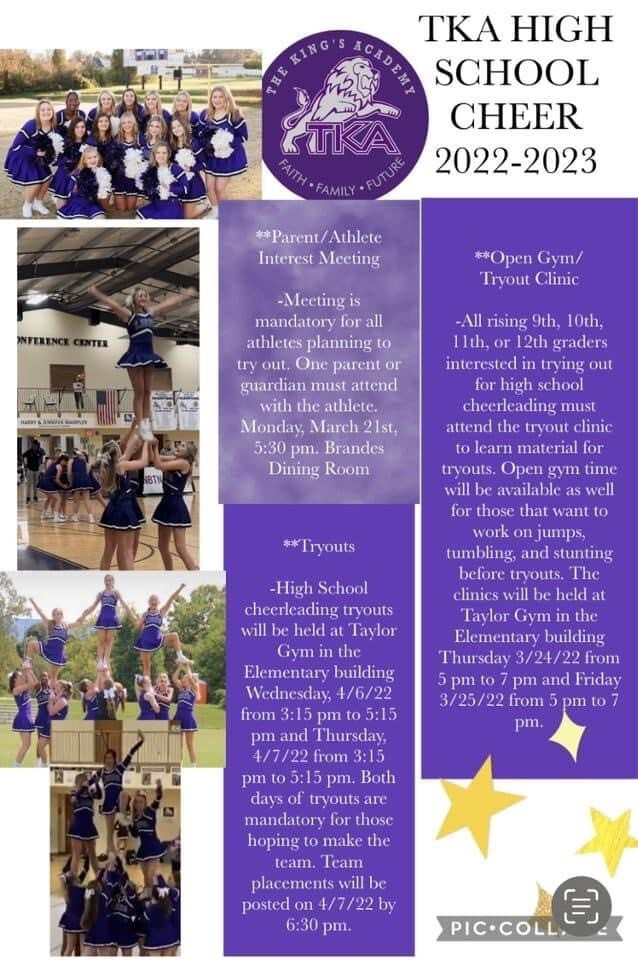table div table+table+table+table+table+table+table+table+table+table+table+table+table+table+table+table+table+table+table+table+table+table+table+table+table div table{width:100%;padding:0}table div table+table+table+table+table+table+table+table+table+table+table+table+table+table+table+table+table+table+table+table+table+table+table+table+table div table img{width:96.23%;padding:0;float:none}table div table+table+table+table+table+table+table+table+table+table+table+table+table+table+table+table+table+table+table+table+table+table+table+table+table div table td{width:100%;padding:0 1.88% 18px}/* styles */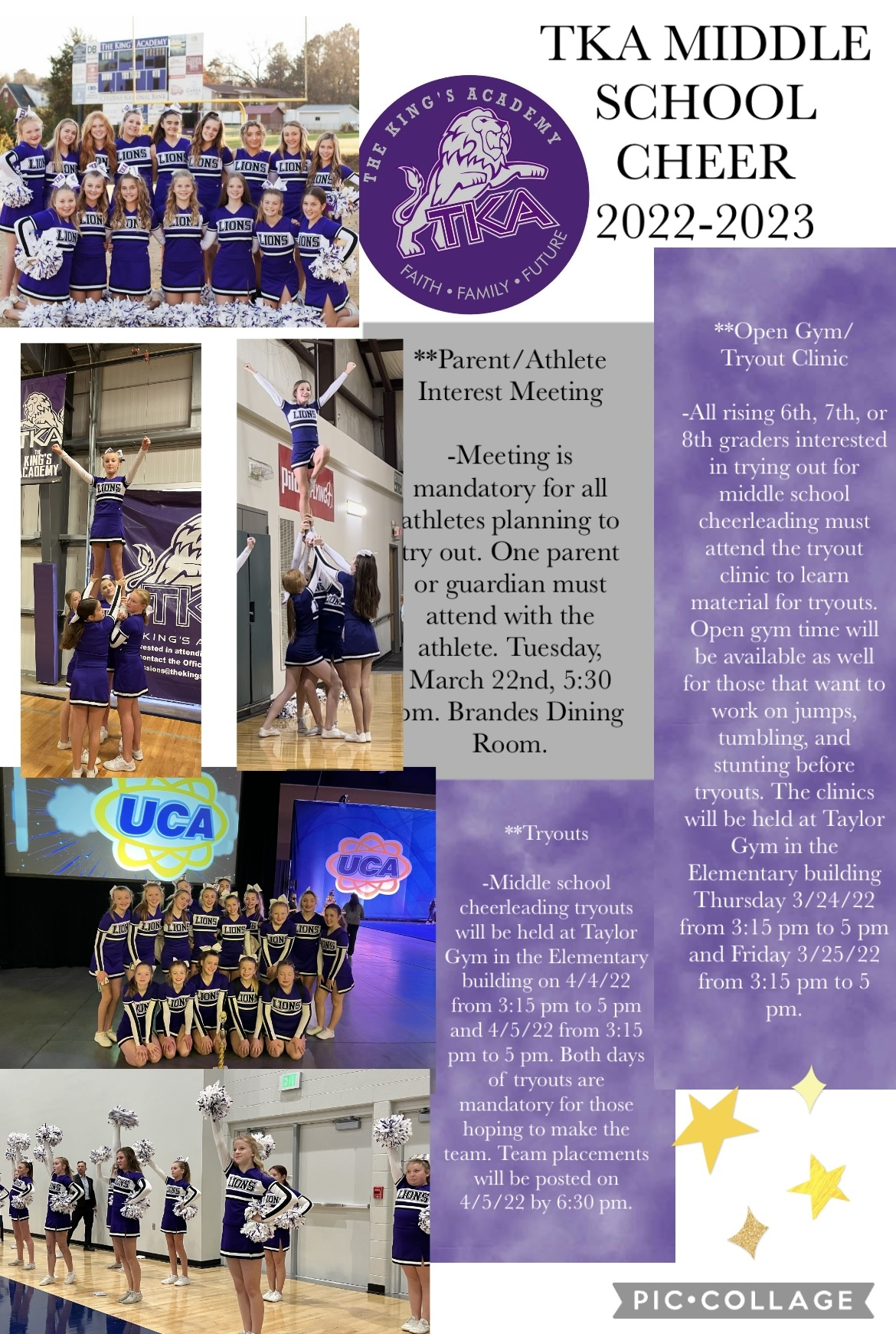table div table+table+table+table+table+table+table+table+table+table+table+table+table+table+table+table+table+table+table+table+table+table+table+table+table+table+table div table{width:100%;padding:0}table div table+table+table+table+table+table+table+table+table+table+table+table+table+table+table+table+table+table+table+table+table+table+table+table+table+table+table div table img{width:96.23%;padding:0;float:none}table div table+table+table+table+table+table+table+table+table+table+table+table+table+table+table+table+table+table+table+table+table+table+table+table+table+table+table div table td{width:100%;padding:0 1.88% 18px}/* styles */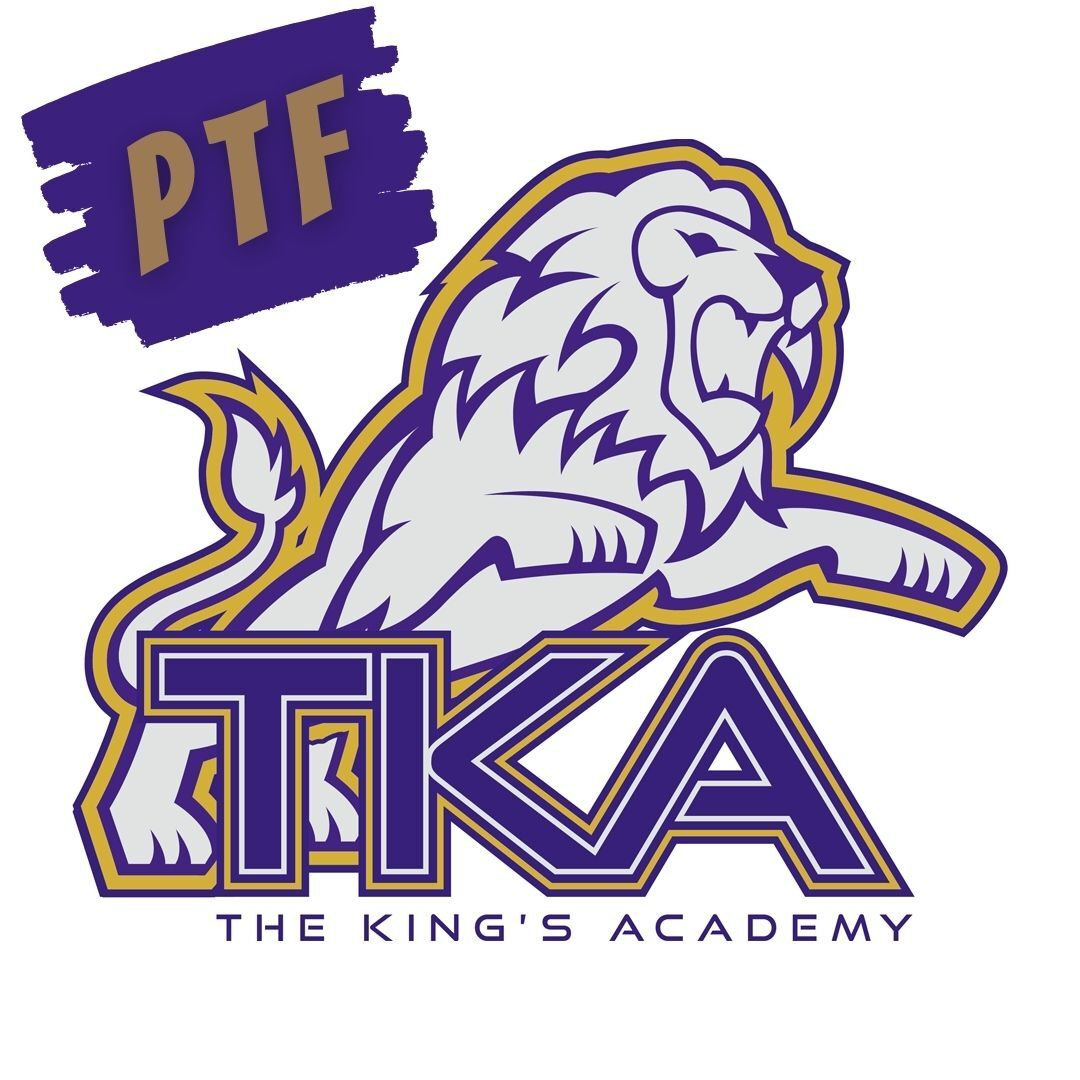# Save these Dates!

Below are the upcoming PTF meetings for the remainder of the year from 3:15 - 4:15 in Brandes:

March 31
April 28
* May 19

PTF will also host a Mother/Son kickball event on Saturday, April 30, at 12:00 p.m. PTF will also host the Family Fun Day on Saturday, May 7, from 10:00 a.m. to 2:00 p.m. More information on these events will come in the days ahead!

 table div table+table+table+table+table+table+table+table+table+table+table+table+table+table+table+table+table+table+table+table+table+table+table+table+table+table+table+table+table div table{width:100%;padding:0}table div table+table+table+table+table+table+table+table+table+table+table+table+table+table+table+table+table+table+table+table+table+table+table+table+table+table+table+table+table div table img{width:96.23%;padding:0;float:none}table div table+table+table+table+table+table+table+table+table+table+table+table+table+table+table+table+table+table+table+table+table+table+table+table+table+table+table+table+table div table td{width:100%;padding:0 1.88% 18px}/* styles */# Elementary Summer Tutoring

Dear TKA Families,

My name is Ms. Addison, and I teach third grade here at TKA. I have been a part of the TKA family for three years now, including a portion of my masters degree internship program. I am excited to announce I will be offering summer tutoring for elementary grades in reading, math, and ELA. I will be offering tutoring to better solidify skills from the grade the student will be leaving, or working on skills to prepare for the upcoming school year. The cost will be \$35/hour which can be made payable through the school. I will be working out of my classroom in the Ogle Elementary building.

Please select the dates and times from the Google from below that you are requesting to sign your student up for. After the deadline of April 8th, 2022, I will organize the requests, finalize a schedule and reach out to each family in regards to your request. Please know space is limited and not all requests will be able to be fulfilled.

 /* styles */ Thank you in advance for the opportunity to be a part of your student's education. I look forward to a fun and successful summer. Ms. Addison 3rd Grade Teacher
 table div table+table+table+table+table+table+table+table+table+table+table+table+table+table+table+table+table+table+table+table+table+table+table+table+table+table+table+table+table+table+table+table+table div table{width:100%;padding:0}table div table+table+table+table+table+table+table+table+table+table+table+table+table+table+table+table+table+table+table+table+table+table+table+table+table+table+table+table+table+table+table+table+table div table img{width:96.23%;padding:0;float:none}table div table+table+table+table+table+table+table+table+table+table+table+table+table+table+table+table+table+table+table+table+table+table+table+table+table+table+table+table+table+table+table+table+table div table td{width:100%;padding:0 1.88% 18px}/* styles */# 27th Annual Golf Tournament

Join us at Egwani Farms on June 13 for our 27th Annual Golf Tournament to benefit TKA's football team!

Gather your company, friends or family and purchase one of the following sponsorships:
- Purple Sponsor includes a 4-person team and a hole sponsorship for \$500
- Silver Sponsor includes an individual player and a hole sponsorship for \$200
- Individual players can participate for \$100

 table div table+table+table+table+table+table+table+table+table+table+table+table+table+table+table+table+table+table+table+table+table+table+table+table+table+table+table+table+table+table+table+table+table+table+table div table{width:100%;padding:0}table div table+table+table+table+table+table+table+table+table+table+table+table+table+table+table+table+table+table+table+table+table+table+table+table+table+table+table+table+table+table+table+table+table+table+table div table img{width:96.23%;padding:0;float:none}table div table+table+table+table+table+table+table+table+table+table+table+table+table+table+table+table+table+table+table+table+table+table+table+table+table+table+table+table+table+table+table+table+table+table+table div table td{width:100%;padding:0 1.88% 18px}/* styles */# Upcoming Sports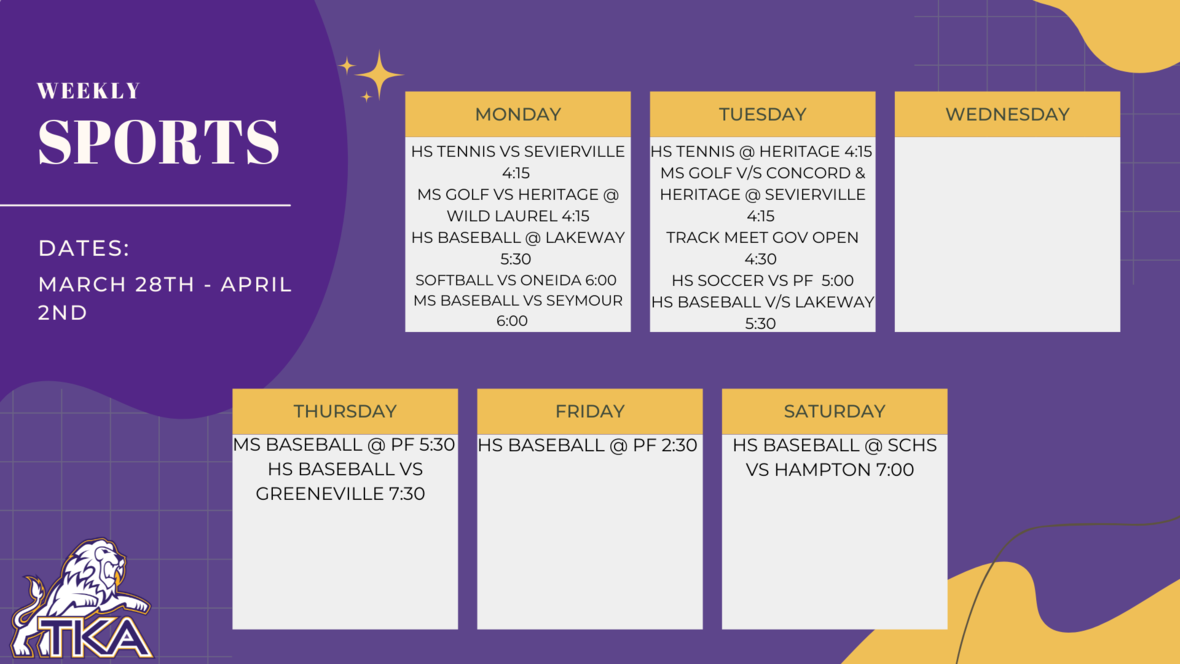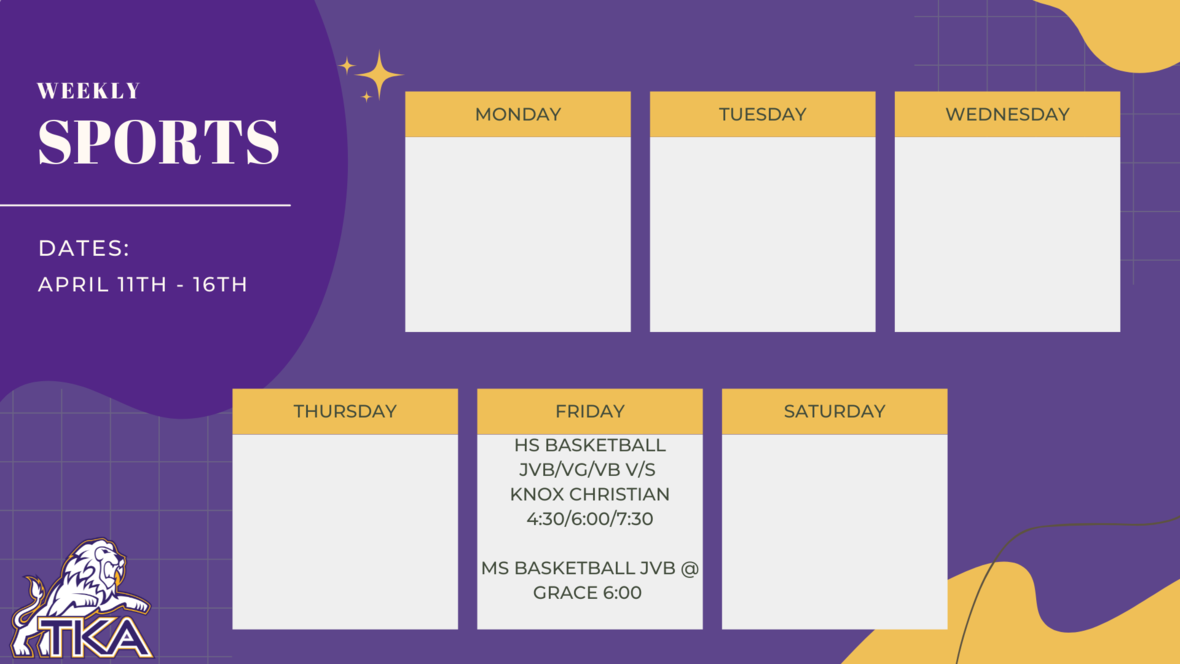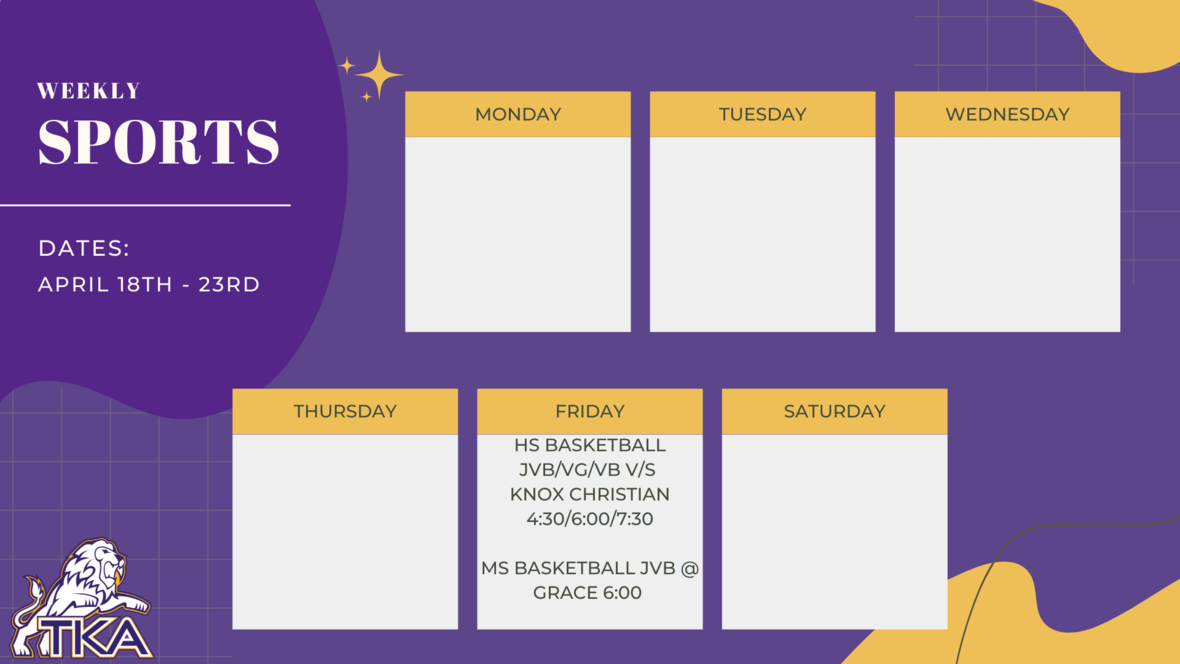table div table+table+table+table+table+table+table+table+table+table+table+table+table+table+table+table+table+table+table+table+table+table+table+table+table+table+table+table+table+table+table+table+table+table+table+table+table+table+table+table+table div table{width:100%;padding:0}table div table+table+table+table+table+table+table+table+table+table+table+table+table+table+table+table+table+table+table+table+table+table+table+table+table+table+table+table+table+table+table+table+table+table+table+table+table+table+table+table+table div table img{width:96.23%;padding:0;float:none}table div table+table+table+table+table+table+table+table+table+table+table+table+table+table+table+table+table+table+table+table+table+table+table+table+table+table+table+table+table+table+table+table+table+table+table+table+table+table+table+table+table div table td{width:100%;padding:0 1.88% 18px}/* styles */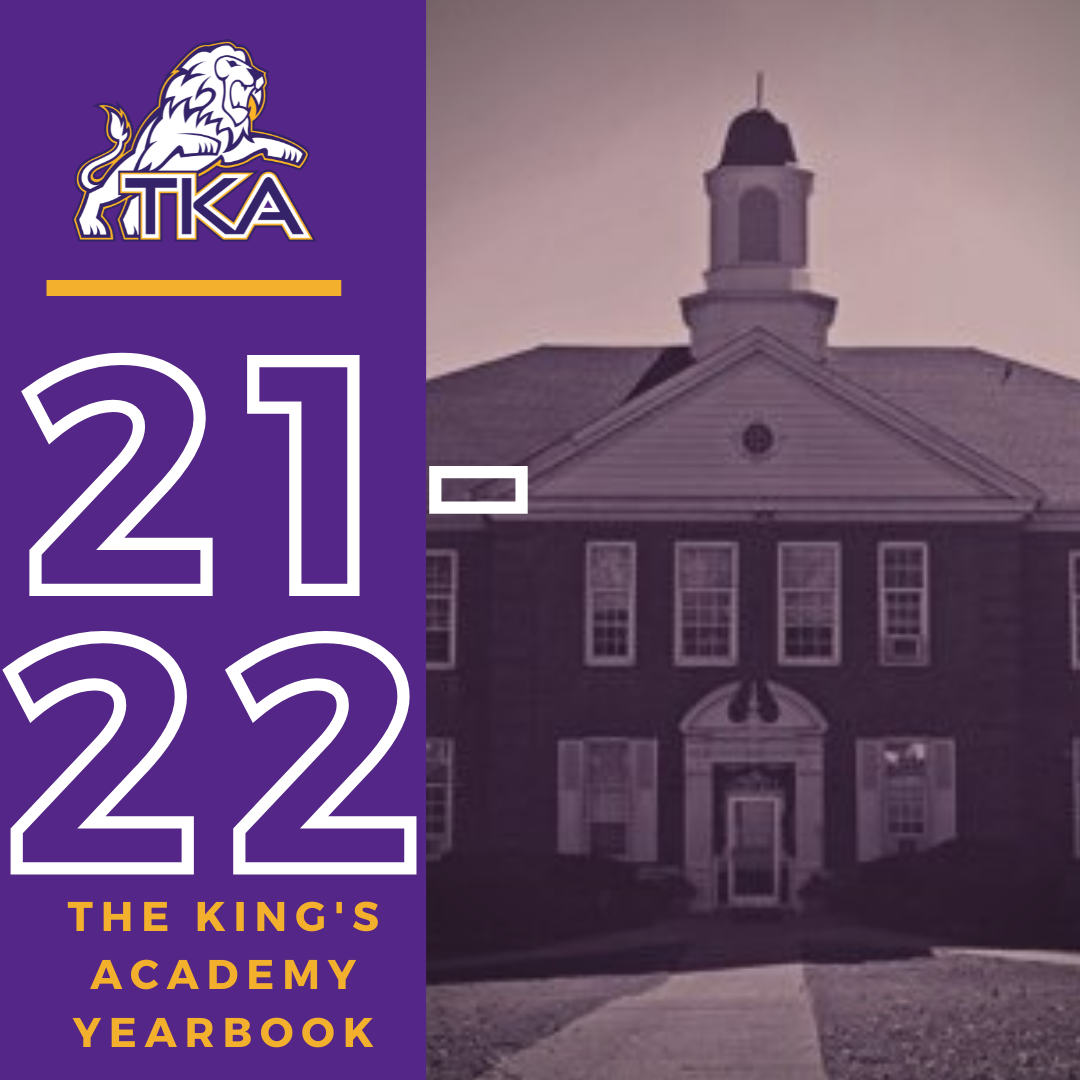# 2021-2022 Yearbook

The price for the 2021-2022 TKA yearbook is \$55. All grades, K3-12 will have the same yearbook. The slight price increase is because we are only going to have one larger all-school book.

All yearbook orders can be placed online--here is the link.Do you have a class of 2022 senior in your household?

Don't miss out on the opportunity to purchase a Senior Tribute for the 2021-2022 yearbook!

Senior Tributes are available for purchase at the bottom of the link listed here.For any questions regarding yearbooks, email Mr. Josh Smith at jsmith@thekingsacademy.net.

 table div table+table+table+table+table+table+table+table+table+table+table+table+table+table+table+table+table+table+table+table+table+table+table+table+table+table+table+table+table+table+table+table+table+table+table+table+table+table+table+table+table+table+table+table+table div table{width:100%;padding:0}table div table+table+table+table+table+table+table+table+table+table+table+table+table+table+table+table+table+table+table+table+table+table+table+table+table+table+table+table+table+table+table+table+table+table+table+table+table+table+table+table+table+table+table+table+table div table img{width:96.23%;padding:0;float:none}table div table+table+table+table+table+table+table+table+table+table+table+table+table+table+table+table+table+table+table+table+table+table+table+table+table+table+table+table+table+table+table+table+table+table+table+table+table+table+table+table+table+table+table+table+table div table td{width:100%;padding:0 1.88% 18px}/* styles */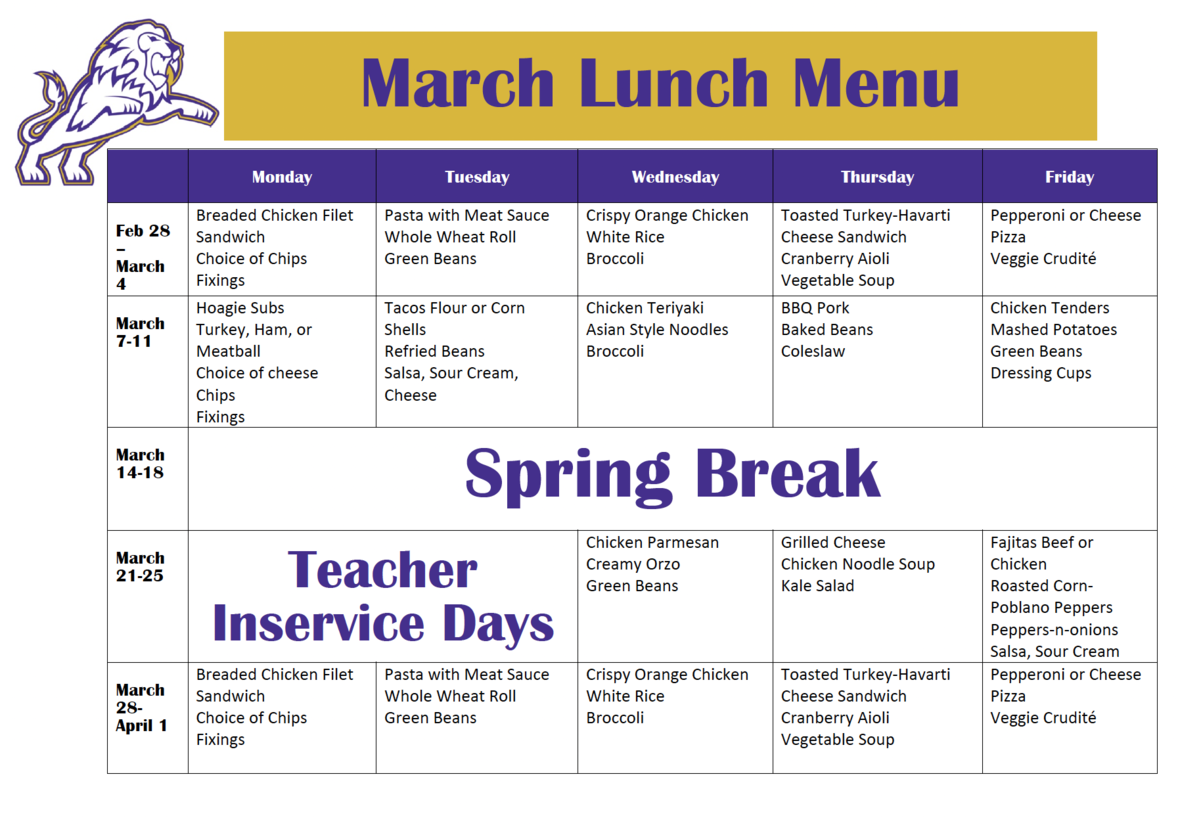table div table+table+table+table+table+table+table+table+table+table+table+table+table+table+table+table+table+table+table+table+table+table+table+table+table+table+table+table+table+table+table+table+table+table+table+table+table+table+table+table+table+table+table+table+table+table+table div table{width:100%;padding:0}table div table+table+table+table+table+table+table+table+table+table+table+table+table+table+table+table+table+table+table+table+table+table+table+table+table+table+table+table+table+table+table+table+table+table+table+table+table+table+table+table+table+table+table+table+table+table+table div table img{width:96.23%;padding:0;float:none}table div table+table+table+table+table+table+table+table+table+table+table+table+table+table+table+table+table+table+table+table+table+table+table+table+table+table+table+table+table+table+table+table+table+table+table+table+table+table+table+table+table+table+table+table+table+table+table div table td{width:100%;padding:0 1.88% 18px}/* styles */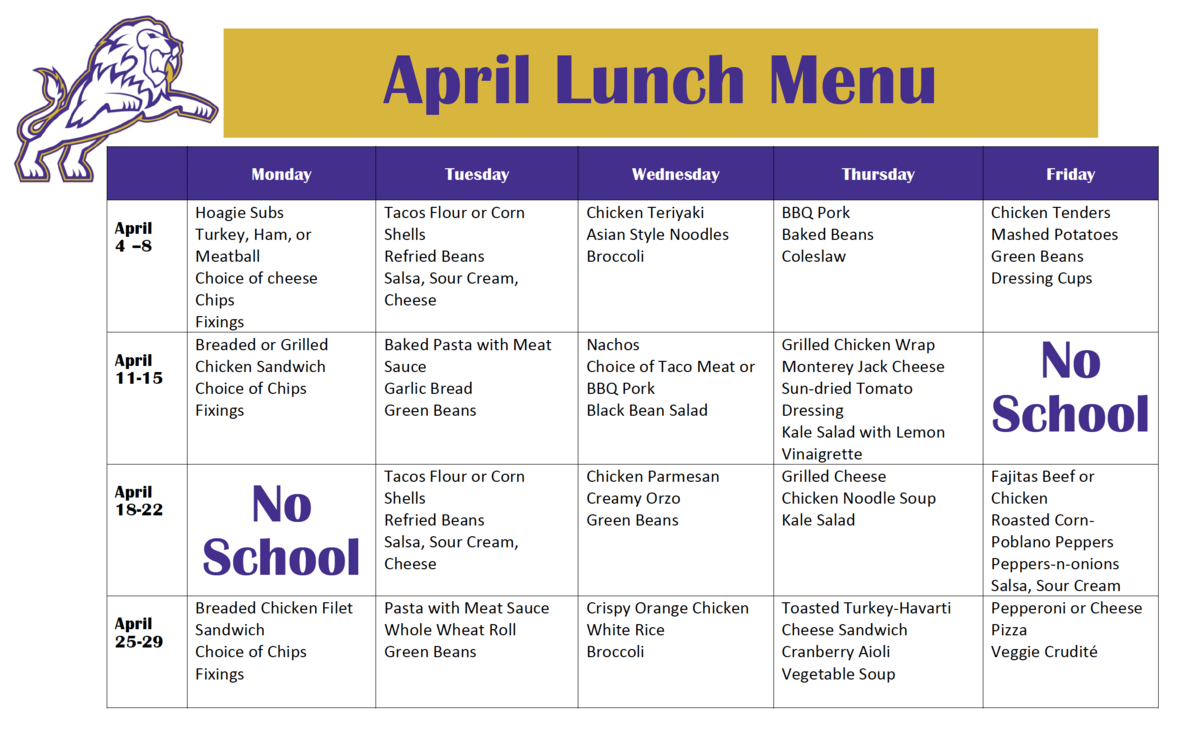table div table+table+table+table+table+table+table+table+table+table+table+table+table+table+table+table+table+table+table+table+table+table+table+table+table+table+table+table+table+table+table+table+table+table+table+table+table+table+table+table+table+table+table+table+table+table+table+table+table div table{width:100%;padding:0}table div table+table+table+table+table+table+table+table+table+table+table+table+table+table+table+table+table+table+table+table+table+table+table+table+table+table+table+table+table+table+table+table+table+table+table+table+table+table+table+table+table+table+table+table+table+table+table+table+table div table img{width:96.23%;padding:0;float:none}table div table+table+table+table+table+table+table+table+table+table+table+table+table+table+table+table+table+table+table+table+table+table+table+table+table+table+table+table+table+table+table+table+table+table+table+table+table+table+table+table+table+table+table+table+table+table+table+table+table div table td{width:100%;padding:0 1.88% 18px}/* styles *//* styles */
 table div table+table+table+table+table+table+table+table+table+table+table+table+table+table+table+table+table+table+table+table+table+table+table+table+table+table+table+table+table+table+table+table+table+table+table+table+table+table+table+table+table+table+table+table+table+table+table+table+table+table+table+table div table{width:100%;padding:0}table div table+table+table+table+table+table+table+table+table+table+table+table+table+table+table+table+table+table+table+table+table+table+table+table+table+table+table+table+table+table+table+table+table+table+table+table+table+table+table+table+table+table+table+table+table+table+table+table+table+table+table+table div table img{width:96.23%;padding:0;float:none}table div table+table+table+table+table+table+table+table+table+table+table+table+table+table+table+table+table+table+table+table+table+table+table+table+table+table+table+table+table+table+table+table+table+table+table+table+table+table+table+table+table+table+table+table+table+table+table+table+table+table+table+table div table td{width:100%;padding:0 1.88% 18px}/* styles */# Covid-19 Procedures

While we are excited to offer in-person education, we must maintain a safe learning environment for all students, faculty/staff, and additional stakeholders.

To do so, we will be working closely with the Sevier County Health Department regarding Covid-19 protocols.

 table div table+table+table+table+table+table+table+table+table+table+table+table+table+table+table+table+table+table+table+table+table+table+table+table+table+table+table+table+table+table+table+table+table+table+table+table+table+table+table+table+table+table+table+table+table+table+table+table+table+table+table+table+table+table div table{width:100%;padding:0}table div table+table+table+table+table+table+table+table+table+table+table+table+table+table+table+table+table+table+table+table+table+table+table+table+table+table+table+table+table+table+table+table+table+table+table+table+table+table+table+table+table+table+table+table+table+table+table+table+table+table+table+table+table+table div table img{width:96.23%;padding:0;float:none}table div table+table+table+table+table+table+table+table+table+table+table+table+table+table+table+table+table+table+table+table+table+table+table+table+table+table+table+table+table+table+table+table+table+table+table+table+table+table+table+table+table+table+table+table+table+table+table+table+table+table+table+table+table+table div table td{width:100%;padding:0 1.88% 18px}/* styles */table div table+table+table+table+table+table+table+table+table+table+table+table+table+table+table+table+table+table+table+table+table+table+table+table+table+table+table+table+table+table+table+table+table+table+table+table+table+table+table+table+table+table+table+table+table+table+table+table+table+table+table+table+table+table+table+table div table{width:100%;padding:0}table div table+table+table+table+table+table+table+table+table+table+table+table+table+table+table+table+table+table+table+table+table+table+table+table+table+table+table+table+table+table+table+table+table+table+table+table+table+table+table+table+table+table+table+table+table+table+table+table+table+table+table+table+table+table+table+table div table img{width:96.23%;padding:0;float:none}table div table+table+table+table+table+table+table+table+table+table+table+table+table+table+table+table+table+table+table+table+table+table+table+table+table+table+table+table+table+table+table+table+table+table+table+table+table+table+table+table+table+table+table+table+table+table+table+table+table+table+table+table+table+table+table+table div table td{width:100%;padding:0 1.88% 18px}/* styles */